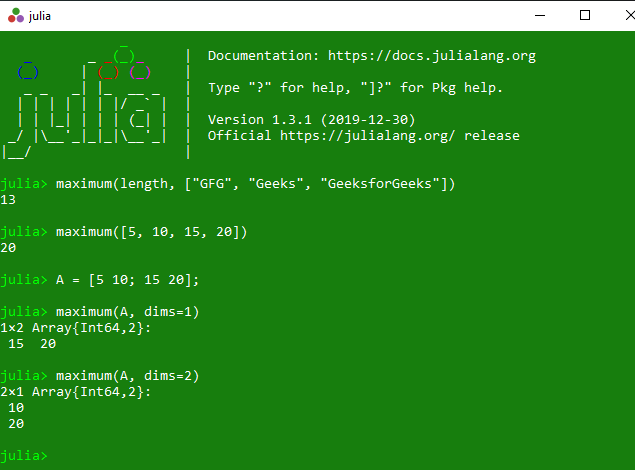# Getting maximum elements in Julia – maximum() and maximum!() Methods

The maximum() is an inbuilt function in julia which is used to return maximum elements in different scenario.

Syntax:
maximum(f, itr)
or
maximum(itr)
or
maximum(A::AbstractArray; dims)

Parameters:

• f: Specified function.
• itr: Specified list of elements.
• A::AbstractArray: Specified array of different dimensions.
• dims: Specified dimensions.

Returns: It returns the maximum elements in different scenario.

Example:

## Julia

 `# Julia program to illustrate ` `# the use of maximum() method`   `# Getting maximum elements in different scenarios`   `# In the below scenario, length function ` `# and a list of elements are used as ` `# parameter. Here the length of string with ` `# maximum alphabets will be returned` `println(maximum(length, [``"GFG"``, ``"Geeks"``, ``"GeeksforGeeks"``]))`   `# In the below scenario, a list of ` `# elements are shown and maximum elements are ` `# returned as output` `println(maximum([``5``, ``10``, ``15``, ``20``]))`   `# Getting the maximum value of an array` `# over the given dimensions` `A ``=` `[``5` `10``; ``15` `20``];` `println(maximum(A, dims ``=` `1``))` `println(maximum(A, dims ``=` `2``))`

Output:The maximum!() is an inbuilt function in julia which is used to calculate the maximum value of the specified array over the specified singleton dimensions.

Syntax: maximum!(r, A)
Parameters:

• r: Specified singleton dimensions.
• A: Specified array.

Returns: It returns the maximum value of the specified array over the specified singleton dimensions.

Example:

## Julia

 `# Julia program to illustrate ` `# the use of maximum !() method`   `# Getting the maximum value of the` `# specified array over the specified` `# singleton dimensions.` `A ``=` `[``5` `10``; ``15` `20``];` `println(maximum !([``1``; ``1``], A))` `println(maximum !([``1` `1``], A))`

Output:

```[10, 20]
[15 20]```

Whether you're preparing for your first job interview or aiming to upskill in this ever-evolving tech landscape, GeeksforGeeks Courses are your key to success. We provide top-quality content at affordable prices, all geared towards accelerating your growth in a time-bound manner. Join the millions we've already empowered, and we're here to do the same for you. Don't miss out - check it out now!